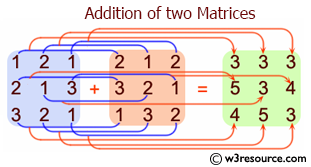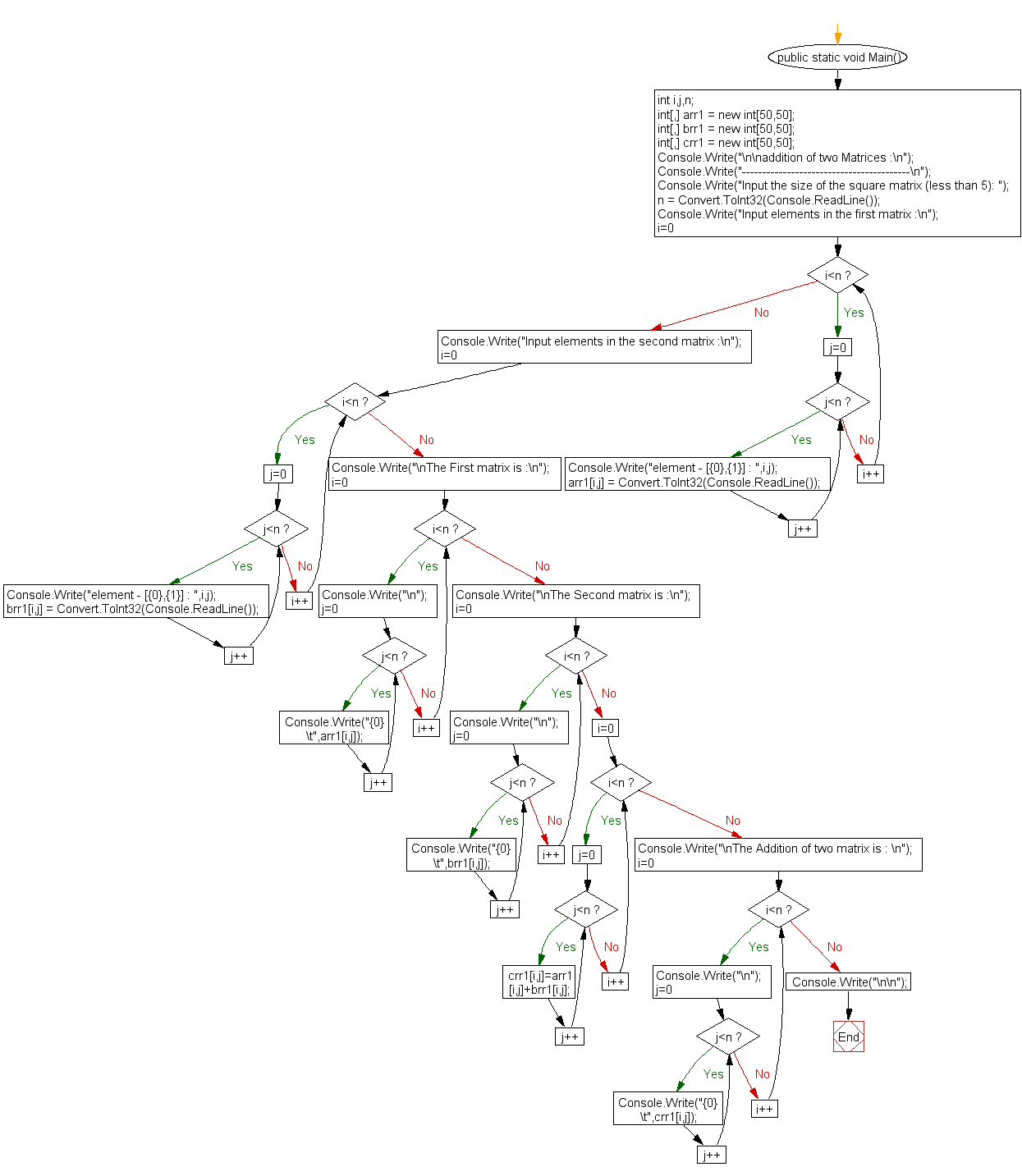﻿ C# Sharp Exercises: Addition of two Matrices - w3resourceC# Sharp Exercises: Addition of two Matrices

C# Sharp Array: Exercise-19 with Solution

Write a program in C# Sharp for addition of two Matrices of same size.Sample Solution:-

C# Sharp Code:

``````using System;
public class Exercise19
{
public static void Main()
{
int i,j,n;
int[,] arr1 = new int[50,50];
int[,] brr1 = new int[50,50];
int[,] crr1 = new int[50,50];

Console.Write("-----------------------------------------\n");
Console.Write("Input the size of the square matrix (less than 5): ");

/* Stored values into the array*/
Console.Write("Input elements in the first matrix :\n");
for(i=0;i<n;i++)
{
for(j=0;j<n;j++)
{
Console.Write("element - [{0},{1}] : ",i,j);
}
}

Console.Write("Input elements in the second matrix :\n");
for(i=0;i<n;i++)
{
for(j=0;j<n;j++)
{
Console.Write("element - [{0},{1}] : ",i,j);
}
}
Console.Write("\nThe First matrix is :\n");
for(i=0;i<n;i++)
{
Console.Write("\n");
for(j=0;j<n;j++)
Console.Write("{0}\t",arr1[i,j]);
}

Console.Write("\nThe Second matrix is :\n");
for(i=0;i<n;i++)
{
Console.Write("\n");
for(j=0;j<n;j++)
Console.Write("{0}\t",brr1[i,j]);
}
/* calculate the sum of the matrix */
for(i=0;i<n;i++)
for(j=0;j<n;j++)
crr1[i,j]=arr1[i,j]+brr1[i,j];
Console.Write("\nThe Addition of two matrix is : \n");
for(i=0;i<n;i++){
Console.Write("\n");
for(j=0;j<n;j++)
Console.Write("{0}\t",crr1[i,j]);
}
Console.Write("\n\n");
}
}
```
```

Sample Output:

```addition of two Matrices :
-----------------------------------------
Input the size of the square matrix (less than 5): 2
Input elements in the first matrix :
element - [0,0] : 2
element - [0,1] : 4
element - [1,0] : 6
element - [1,1] : 8
Input elements in the second matrix :
element - [0,0] : 1
element - [0,1] : 3
element - [1,0] : 5
element - [1,1] : 7
The First matrix is :
2       4
6       8
The Second matrix is :
1       3
5       7
The Addition of two matrix is :
3       7
11      15
```

Flowchart:C# Sharp Code Editor: